# module utils.orttraining_helper#

## Short summary#

module onnxcustom.utils.orttraining_helper

ONNX manipulations to help build ONNX gradient graphs.

source on GitHub

## Functions#

function

truncated documentation

_loss_elastic

Implements mixture of losses l1 and l2.

_loss_l1

Implements loss l1.

_loss_l2

Implements loss l2.

_loss_log

This only works for a binary classification. The log loss is ‘log(yt, yp) = (1-yt)log(1-yp) - ytlog(yp), this …

_rewrite_op_no_grad

_unique_name

Returns a name different from any name in existing_names.

add_loss_output

Modifies an ONNX graph to add operators to score and allow training.

get_train_initializer

Returns the list of initializers to train.

penalty_loss_onnx

Returns onnx nodes to compute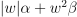where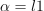and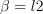.

## Documentation#

ONNX manipulations to help build ONNX gradient graphs.

source on GitHub

onnxcustom.utils.orttraining_helper._loss_elastic(existing_names, elem, shape, output_name, label_name, weight_name, loss_name, l1_weight=0.5, l2_weight=0.5)#

Implements mixture of losses l1 and l2.

source on GitHub

onnxcustom.utils.orttraining_helper._loss_l1(existing_names, elem, shape, output_name, label_name, weight_name, loss_name)#

Implements loss l1.

source on GitHub

onnxcustom.utils.orttraining_helper._loss_l2(existing_names, elem, shape, output_name, label_name, weight_name, loss_name)#

Implements loss l2.

source on GitHub

onnxcustom.utils.orttraining_helper._loss_log(existing_names, elem, shape, output_name, label_name, weight_name, loss_name, eps=1e-06)#

This only works for a binary classification. The log loss is ‘log(yt, yp) = (1-yt)log(1-yp) - ytlog(yp), this only works for a binary classification where yp is the predicted probability, yt is the expected probability. yt is expected to be binary, yp is a matrix with two columns, the sum on every line is 1. Parameter eps is used to avoid computing log(0).

source on GitHub

source on GitHub

onnxcustom.utils.orttraining_helper._unique_name(existing_names, name)#

Returns a name different from any name in existing_names.

Parameters:
• existing_names – set of names

• name – current

Returns:

unique name

source on GitHub

onnxcustom.utils.orttraining_helper.add_loss_output(onx, score_name='squared_error', loss_name='loss', label_name='label', weight_name=None, penalty=None, output_index=None, **kwargs)#

Modifies an ONNX graph to add operators to score and allow training.

Parameters:
• onx – onx graph

• score_name – name of the score

• loss_name – name of the output loss

• label_name – name of the label input

• weight_name – None or any value to consider weight while computing loss

• penalty – dictionary similar to the following one { weight_name: {‘l1’: alpha, ‘l2’: beta} } or { weight_name: beta}, it adds a L1 and/or L2 penalty to one input or initializer, penalty =• output_index – the output used to compute the loss, if None, the function assumes there is only one output, it must be specified if there are more than 1, it can be an integer or a string (output name)

• kwargs – additional arguments for losses (see below)

Returns:

modified graph

Possible values for score_name:

• ‘squared_error’ or ‘l2’: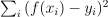or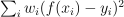if weight_name is not None

• ‘absolute_error’ or ‘l1’: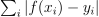or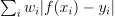if weight_name is not None

• ‘elastic’: mixture of losses, kwargs must define l1_weight and l2_weight, undefined, default value are 0.5

• ‘log’: log loss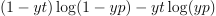,

this only works for a binary classification where yp is the predicted probability, yt is the expected probability. yt is expected to be binary, yp is a matrix with two columns, the sum on every line is 1.

See example Train a scikit-learn neural network with onnxruntime-training on GPU. Next example shows the loss with L1 and L2 loss.

Next example shows how to add a L2 loss with L1 and L2 penalties on the coefficients.

source on GitHub

onnxcustom.utils.orttraining_helper.get_train_initializer(onx)#

Returns the list of initializers to train.

Returns:

dictionary {name: (value, tensor)}

The function walk through the list of initializers and returns all tensors with elements from types float or double.

source on GitHub

onnxcustom.utils.orttraining_helper.penalty_loss_onnx(name, dtype, l1=None, l2=None, existing_names=None)#

Returns onnx nodes to computewhereand.

Parameters:
• name – name of weights

• dtype – numpy dtype

• l1 – coefficient for L1 norm

• l2 – coefficient for L2 norm

• existing_names – names already taken in the ONNX graph

Returns:

initializer, nodes

source on GitHub